﻿ On The Hermite- Hadamard-Fejér Type Integral Inequality for Convex Function

### On The Hermite- Hadamard-Fejér Type Integral Inequality for Convex Function

Mehmet Zeki Sarikaya, Samet ErdenOPEN ACCESSPEER-REVIEWED

## On The Hermite- Hadamard-Fejér Type Integral Inequality for Convex Function

Mehmet Zeki Sarikaya1,, Samet Erden2

1Department of Mathematics, Faculty of Science and Arts, Düzce University, Konu-ralp Campus, Düzce-TURKEY

2Department of Mathematics, Faculty of Science, Bartn University, Konuralp Cam-pus, BARTIN-TURKEY

### Abstract

In this paper, we extend some estimates of the right hand side of a Hermite- Hadamard-Fejér type inequality for functions whose first derivatives absolute values are convex. The results presented here would provide extensions of those given in earlier works.

• Sarikaya, Mehmet Zeki, and Samet Erden. "On The Hermite- Hadamard-Fejér Type Integral Inequality for Convex Function." Turkish Journal of Analysis and Number Theory 2.3 (2014): 85-89.
• Sarikaya, M. Z. , & Erden, S. (2014). On The Hermite- Hadamard-Fejér Type Integral Inequality for Convex Function. Turkish Journal of Analysis and Number Theory, 2(3), 85-89.
• Sarikaya, Mehmet Zeki, and Samet Erden. "On The Hermite- Hadamard-Fejér Type Integral Inequality for Convex Function." Turkish Journal of Analysis and Number Theory 2, no. 3 (2014): 85-89.

 Import into BibTeX Import into EndNote Import into RefMan Import into RefWorks

### 1. Introduction

Definition 1. The function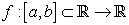, is said to be convex if the following inequality holds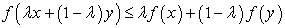for all x,y∈[a,b] and λ∈ [0,1]. We say that f is concave if (-f) is convex.

The following inequality is well known in the literature as the Hermite-Hadamard integral inequality (see, [4, 10]):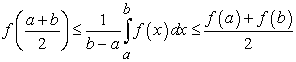(1.1)

where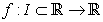is a convex function on the interval I of real numbers and a, b ∈ I with a < b.

In , Dragomir and Agarwal proved the following results connected with the right part of (1.1).

Lemma 1. Let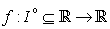be a differentiable mapping on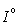,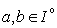with a < b. If f’ ∈ L[a,b], then the following equality holds: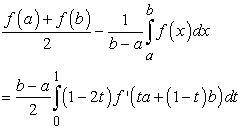(1.2)

Theorem 1. Letbe a differentiable mapping on,with a < b. If |f’| is convex on [a,b], then the following inequality holds: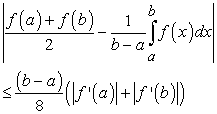(1.3)

Theorem 2. Letbe a differentiable mapping on,with a < b; f’ ∈ L(a,b) and p > 1. If the mapping |f’|p/(p-1) is convex on [a,b], then the following inequality holds: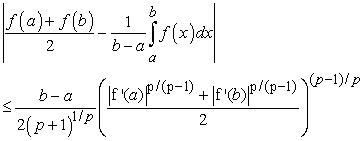(1.4)

The most well-known inequalities related to the integral mean of a convex function are the Hermite Hadamard inequalities or its weighted versions, the so-called Hermite- Hadamard- Fejér inequalities (see, [8, 13, 14, 15, 16, 19, 20]). In , Fejer gave a weighted generalizatinon of the inequalities (1.1) as the following:

Theorem 3.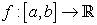, be a convex function, then the inequality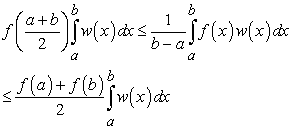(1.5)

holds, where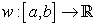is nonnegative, integrable, and symmetric about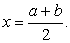In , some inequalities of Hermite-Hadamard-Fejer type for differentiable convex mappings were proved using the following lemma.

Lemma 2. Let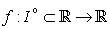be a differentiable mapping on,with a < b, and w: [a,b]→[0,∞) be a differentiable mapping. If f’ ∈L[a,b], then the following equality holds: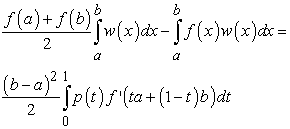(1.6)

for each t 2 [0,1]; where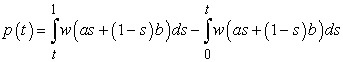The main result in  is as follows:

Theorem 4. Letbe a differentiable mapping on,with a < b, and w: [a,b]→[0,∞) be a differentiable mapping and symmetric to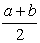. If |f’| is convex on [a,b] ; then the following inequality holds: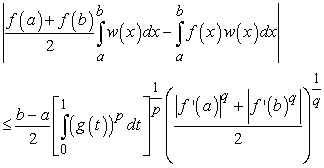(1.7)

where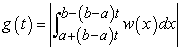for t∈ [0,1].

Definition 2. Let f∈L1[a,b]. The Riemann-Liouville integrals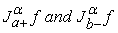of order α > 0 with a≥ 0 are defined by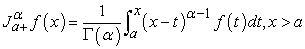And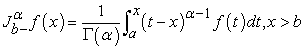respectively. Here,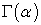is the Gamma function and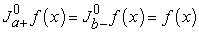.

Meanwhile, Sarikaya et al.  presented the following important integral identity including the first-order derivative of f to establish many interesting Hermite-Hadamard type inequalities for convexity functions via Riemann-Liouville fractional integrals of the order α > 0.

Lemma 3. Letbe a differentiable mapping on (a,b) with a < b. If f’∈L[a,b], then the following equality for fractional integrals holds: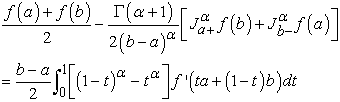(1.8)

It is remarkable that Sarikaya et al.  first give the following interesting integral inequalities of Hermite-Hadamard type involving Riemann-Liouville fractional

Theorem 5. Letbe a positive function with 0 ≤ a < b and f∈L1 [a,b]. If f is a convex function on [a,b], then the following inequalities for fractional integrals hold: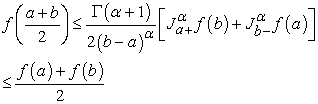(1.9)

with α > 0:

For some recent results connected with fractional integral inequalities see [1, 2, 8, 17, 18].

In this article, using functions whose derivatives absolute values are convex, we obtained new inequalities of Hermite-Hadamard-Fejer type and Hermite-Hadamard type involving fractional integrals. The results presented here would provide extensions of those given in earlier works.

### 2. Main Results

We will establish some new results connected with the right-hand side of (1.5) and (1.1) involving fractional integrals used the following Lemma. Now, we give the following new Lemma for our results:

Lemma 4. Letbe a differentiable mapping on,with a < b and let. If f’, w∈L[a,b], then, for all x∈ [a,b], the following equality holds: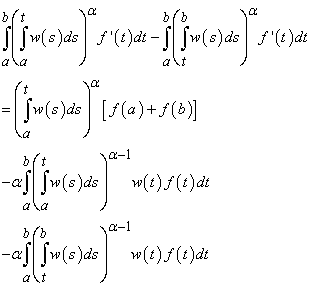(2.1)

where α > 1:

Proof. By integration by parts, we have the following equalities: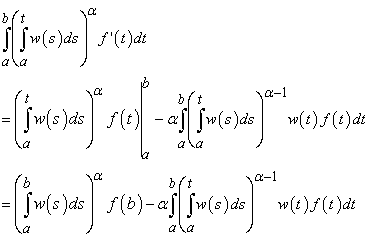(2.2)

and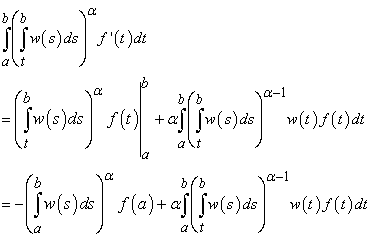(2.3)

Subtracting (2.3) from (2.2), we obtain (2.1). This completes the proof.

Remark 1. If we take w(s) = 1 in 2.1; the identity (2.1) reduces to the identity (1.8).

Corollary 1. Under the same assumptions of Lemma 4 with α = 1; then the following identity holds: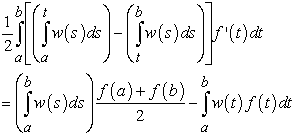(2.4)

Remark 2. If we take w(s) = 1 in (2.4), the identity (2.4) reduces to the identity (1.2).

Now, by using the above lemma, we prove our main theorems:

Theorem 6. Letbe a differentiable mapping on,with a < b and letbe continuous on [a,b]. If |f’| is convex on [a,b], then the following inequality holds: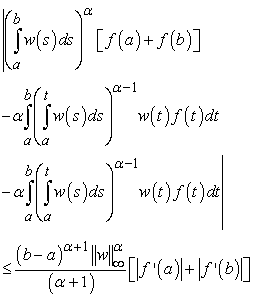where α > 0 and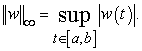Proof. We take absolute value of (2.1), we find that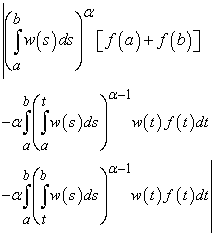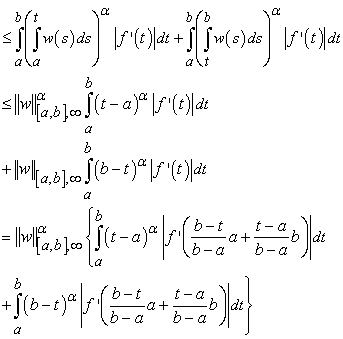Since |f’| is convex on [a,b], it follows that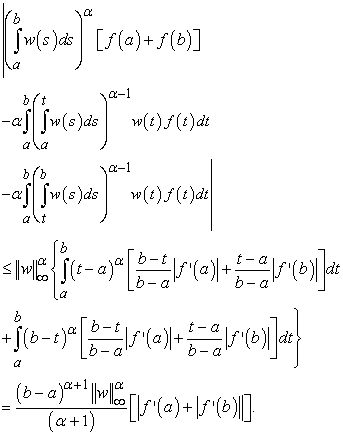Hence, the proof of theorem is completed.

Corollary 2. Under the same assumptions of Theorem 6 with w(s) = 1, then the following inequality holds: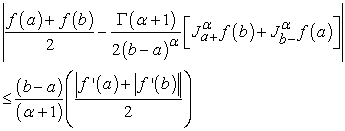(2.5)

Proof. This proof is given by Sarikaya et. al in .

Remark 3. If we take α = 1 in (2.5); the inequality (2.5) reduces to (1.3).

Corollary 3. Under the same assumptions of Theorem 6 with α = 1, then the following inequality holds: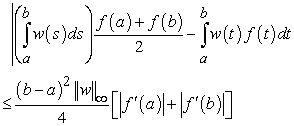Theorem 7. Letbe a differentiable mapping on,with a < b and letbe continuous on [a,b]. If |f’|q is convex on [a,b], q > 1, then the following inequality holds: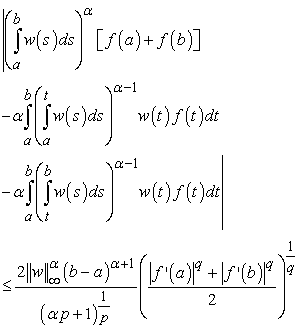(2.6)

where α > 0,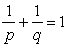, and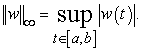Proof. We take absolute value of (2.1). Using Holder’s inequality, we find that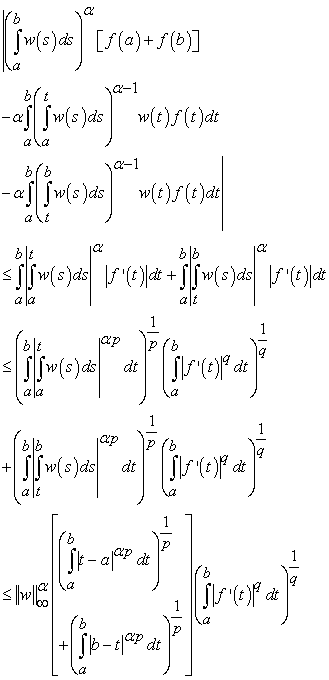Since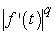is convex on [a,b]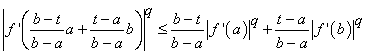(2.7)

From (2.7), it follows that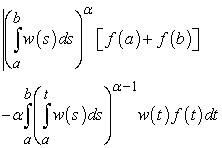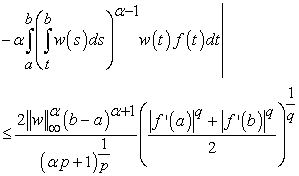which this completes the proof.

Corollary 4. Under the same assumptions of Theorem 6 with w(s) = 1, then the following inequality holds: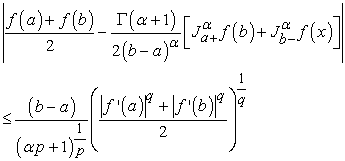(2.8)

Corollary 5. Let the conditions of Theorem 7 hold. If we take α = 1 in (2.6), then the following inequality holds: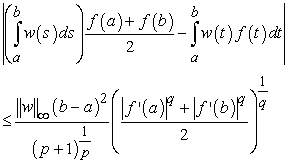Remark 4. If we take w(s) = 1 in (2.9), we have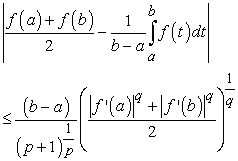which is proved by Dragomir and Agarwal in .

### References

  Z. Dahmani, On Minkowski and Hermite-Hadamard integral inequalities via fractional integration, Ann. Funct. Anal. 1 (1) (2010), 51-58.In article CrossRef  J. Deng and J. Wang, Fractional Hermite-Hadamard inequalities for (α; m)-logarithmically convex functions.In article  S. S. Dragomir and R.P. Agarwal, Two inequalities for differentiable mappings and applications to special means of real numbers and to trapezoidal formula, Appl. Math. lett., 11 (5) (1998), 91-95.In article CrossRef  S. S. Dragomir and C. E. M. Pearce, Selected Topics on Hermite-Hadamard Inequalities and Applications, RGMIA Monographs, Victoria University, 2000.In article  S. Hussain, M.A. Latif and M. Alomari, Generalized duble-integral Ostrowski type inequalities on time scales, Appl. Math. Letters, 24 (2011), 1461-1467.In article CrossRef  M. E. Kiris and M. Z. Sarikaya, On the new generalization of Ostrowski type inequality for double integrals, International Journal of Modern Mathematical Sciences, 2014, 9 (3): 221-229.In article  L. Fejer, Über die Fourierreihen, II. Math. Naturwiss. Anz Ungar. Akad. Wiss., 24 (1906), 369.390. (Hungarian).In article  I. I, scan, Hermite-Hadamard-Fejer type inequalities for convex functions via fractional integrals, arXiv preprint arXiv: 1404. 7722 (2014).In article  U.S. Krmac, Inequalities for dif ferentiable mappings and applications to special means of real numbers and to midpoint formula, Appl. Math. Comp., 147 (2004), 137-146.In article CrossRef  J. Peµcari´c, F. Proschan and Y.L. Tong, Convex functions, partial ordering and statistical applications, Academic Press, New York, 1991.In article  M. Z. Sarikaya, E. Set, H. Yaldiz and N., Basak, Hermite -Hadamard.s inequalities for fractional integrals and related fractional inequalities, Mathematical and Computer Modelling, 57 (2013) 2403. 2407.In article  M. Z. Sarikaya and H. Yildirim, On Hermite-Hadamard type inequalities for Riemann- Liouville fractional integrals, SubmitedIn article  M. Z. Sarikaya, On new Hermite Hadamard Fejer Type integral inequalities, Studia Universitatis Babes-Bolyai Mathematica., 57 (2012), No. 3, 377-386.In article  K-L. Tseng, G-S. Yang and K-C. Hsu, Some inequalities for differentiable mappings and applications to Fejer inequality and weighted trapozidal formula, Taiwanese J. Math. 15 (4), pp: 1737-1747, 2011.In article  C.-L. Wang, X.-H. Wang, On an extension of Hadamard inequality for convex functions, Chin. Ann. Math. 3 (1982) 567. 570.In article  S.-H. Wu, On the weighted generalization of the Hermite-Hadamard inequality and its applications, The Rocky Mountain J. of Math., vol. 39, no. 5, pp. 1741. 1749, 2009.In article  M. Tunc, On new inequalities for h-convex functions via Riemann-Liouville fractional integration, Filomat 27: 4 (2013), 559. 565.In article  J. Wang, X. Li, M. Feckan and Y. Zhou, Hermite-Hadamard-type inequalities for Riemann-Liouville fractional integrals via two kinds of convexity, Appl. Anal. (2012).In article  B-Y, Xi and F. Qi, Some Hermite-Hadamard type inequalities for differentiable convex func- tions and applications, Hacet. J. Math. Stat. 42 (3), 243. 257 (2013).In article  B-Y, Xi and F. Qi, Hermite-Hadamard type inequalities for functions whose derivatives are of convexities, Nonlinear Funct. Anal. Appl. 18 (2), 163. 176 (2013).In article  Y. Zhang and J-R. Wang, On some new Hermite-Hadamard inequalities involving Riemann-Liouville fractional integrals, Journal of Inequalities and Applications 2013, 2013: 220.In article